# Math in Focus Grade 8 Chapter 4 Review Test Answer Key

Go through the Math in Focus Grade 8 Workbook Answer Key Chapter 4 Review Test to finish your assignments.

## Math in Focus Grade 8 Course 3 A Chapter 4 Review Test Answer Key

Concepts and Skills

Find the slope of each line using the points indicated. Then write an equation for it.

Question 1.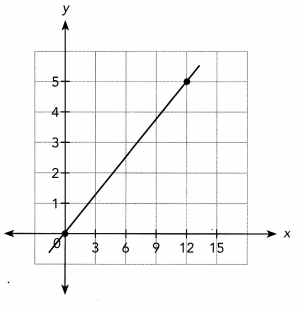By seeing the above graph we can say that the line passes through (0, 0) and (12,5)
slope = (5 – 0)/(12-0)
slope = 5/12
The line passes through the y-axis at the point (0, 0).
Thus m = 5/12 and y-intercept b is 0.
y = 5/12 x

Question 2.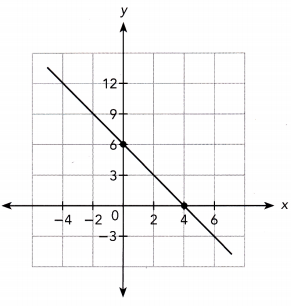The line passes through the points (4, 0) and (0, 6).
Slope m = $$\frac{6-0}{0-4}$$
= $$\frac{6}{-4}$$
= –$$\frac{3}{2}$$
The line passes through the y-axis at the point (0, 6).
Thus m = –$$\frac{3}{2}$$ and y-intercept b is 6.
y = –$$\frac{3}{2}$$x + 6

Question 3.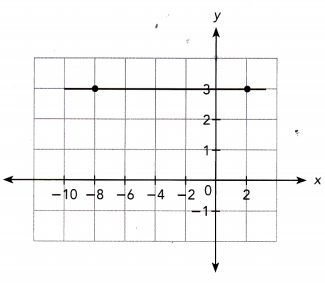The line passes through the points (2, 3) and (-8, 3).
Slope m = $$\frac{3-3}{-8-2}$$
= $$\frac{0}{-10}$$
= 0
The line passes through the y-axis at the point (0, 3).
Thus m = 0 and y-intercept b is 3.
y = 3

Question 4.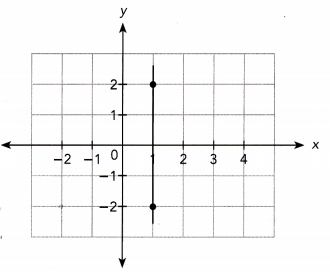The line passes through the points (1, 2) and (1, -2).
Slope m = $$\frac{-2-2}{1-1}$$
undefined

For each line, state its slope and its y-intercept.

Question 5.
y = $$\frac{1}{2}$$x – 3
The equation is in the form of y = mx + b
y = $$\frac{1}{2}$$x – 3
m = $$\frac{1}{2}$$
y-intercept = -3

Question 6.
y = -3x + 4
The equation is in the form of y = mx + b
y = -3x + 4
m = -3 and
y-intercept = 4

Write an equation of each line given its slope and its y-intercept.

Question 7.
Slope, m = -4
y-intercept, b = –$$\frac{1}{3}$$
Given,
Slope, m = -4
y-intercept, b = –$$\frac{1}{3}$$
y = -4x – $$\frac{1}{3}$$

Question 8.
Slope, m = $$\frac{2}{5}$$
y-intercept, b = 3
Given,
Slope, m = $$\frac{2}{5}$$
y-intercept, b = 3
y = $$\frac{2}{5}$$x + 3

Question 9.
Write an equation of the line parallel to 5y = 3x + 12 that has a y-intercept of 2.
Given,
5y = 3x + 12
y = $$\frac{3}{5}$$x + 12
y-intercept of 2
y = $$\frac{3}{5}$$x + 2

Question 10.
Write an equation of the line that has slope –$$\frac{1}{2}$$ and passes through the point (-4, 5).
Given,
slope m = –$$\frac{1}{2}$$
y-intercept = 5
The equation of the line is y = mx + b
y = –$$\frac{1}{2}$$x + 5

Question 11.
Write an equation of the line that passes through the point (-4, -4) and is parallel to 2y – x = -6.
Given equation
2y – x = -6
2y = x – 6
y = $$\frac{1}{2}$$x – 3
the line that passes through the point (-4, -4)
y = $$\frac{1}{2}$$x – 4

Question 12.
Write an equation of the line that passes through the point (-4, -3) and is parallel to 4y – x = -16.
Given equation
4y – x = -16
4y = x – 16
y = $$\frac{1}{4}$$x – 4
the line that passes through the point (-4, -3)
y = $$\frac{1}{4}$$x – 3

Write an equation of the line that passes through each pair of points.

Question 13.
(0, 0) and (7, 7)
The line passes through the points (0, 0) and (7, 7).
Slope m = $$\frac{7-0}{7-0}$$
= $$\frac{7}{7}$$
= 1
The line passes through the y-axis at the point (0, 0).
Thus m = 1 and y-intercept b is 0.
y = x

Question 14.
(1, 2) and (4, 8)
The line passes through the points (1, 2) and (4, 8).
Slope m = $$\frac{8-2}{4-1}$$
= $$\frac{6}{3}$$
= 2
Thus m = 2 and y-intercept b is 0.
y = 2x

Use graph paper. Graph each linear equation. Use 1 grid square on both axes to represent 1 unit for the interval from -5 to 5.

Question 15.
4y = -3x – 8
Given the equation
4y = -3x – 8
y = –$$\frac{3}{4}$$x – 2
slope = –$$\frac{3}{4}$$ and
y-intercept = -2

Question 16.
Slope = $$\frac{1}{3}$$; (0, -2)
Given,
Slope = $$\frac{1}{3}$$; (0, -2)
The equation of the line is y = mx + b
Substitute the given slope and points in the equation.
The equation of the line is y = $$\frac{1}{3}$$x – 2

Problem Solving

Question 17.
Landscaping Company A and Company B each charges a certain amount, C dollars, as consultation fee, plus a fixed hourly charge.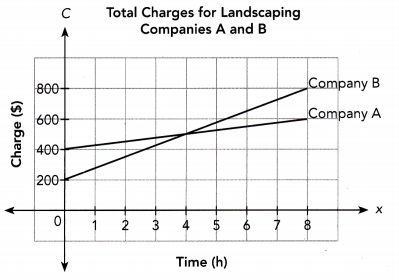a) Find the amount each landscaping company charges as its consultation fee.
Answer: Company A charges $400 and Company B charges$200.

b) Which company charges a greater amount per hour?

Question 18.
The operator of a charter bus service charges a certain amount for a bus, plus per-passenger charge. The graph shows the total charges, C dollars, for carrying x passengers.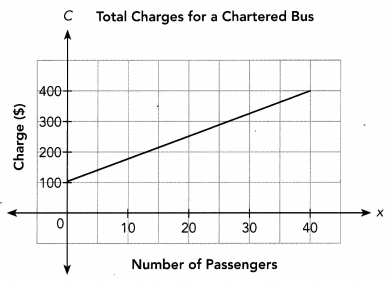a) Find the vertical intercept and explain what information it gives about the situation.
Answer: The vertical intercept is 100

b) Find the slope of the graph and explain what information it gives about the situation.